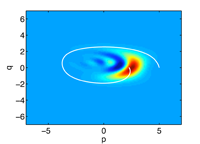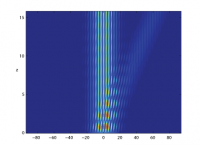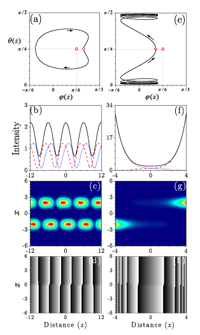October 2010
Mon Tue Wed Thu Fri Sat Sun
« Sep   Nov »
123
45678910
11121314151617
18192021222324
25262728293031

## Quantum tunneling as a classical anomaly

Carl M. Bender, Daniel W. Hook

Classical mechanics is a singular theory in that real-energy classical particles can never enter classically forbidden regions. However, if one regulates classical mechanics by allowing the energy E of a particle to be complex, the particle exhibits quantum-like behavior: Complex-energy classical particles can travel between classically allowed regions separated by potential barriers. When Im(E) -> 0, the classical tunneling probabilities persist. Hence, one can interpret quantum tunneling as an anomaly. A numerical comparison of complex classical tunneling probabilities with quantum tunneling probabilities leads to the conjecture that as ReE increases, complex classical tunneling probabilities approach the corresponding quantum probabilities. Thus, this work attempts to generalize the Bohr correspondence principle from classically allowed to classically forbidden regions.

http://arxiv.org/abs/1011.0121
High Energy Physics – Theory (hep-th); Mathematical Physics (math-ph); Quantum Physics (quant-ph)

## Probing the small distance structure of canonical quantum gravity using the conformal group

Gerard ‘t Hooft

In canonical quantum gravity, the formal functional integral includes an integration over the local conformal factor, and we propose to perform the functional integral over this factor before doing any of the other functional integrals. By construction, the resulting effective theory would be expected to be conformally invariant and therefore finite. However, also the conformal integral itself diverges, and the effects of a renormalization counter term are considered. It generates problems such as unitarity violation, due to a Landau-like ghost, and conformal anomalies. Adding (massive or massless) matter fields does not change the picture. Various alternative ideas are offered, including a more daring speculation, which is that no counter term should be allowed for at all. This has far-reaching and important consequences, which we discuss. A surprising picture emerges of quantized elementary particles interacting with a gravitational field, in particular gravitons, which are “partly classical”. This approach was inspired by a search towards the reconciliation of Hawking radiation with unitarity and locality, and it offers basic new insights there.
Comments: 22 pages (incl. title page), 1 figure. Substantial changes in the discussion sections, minor errors corrected, and references added

http://arxiv.org/abs/1009.0669
General Relativity and Quantum Cosmology (gr-qc); High Energy Physics – Theory (hep-th)

## Wave packet evolution in non-Hermitian quantum systems

Eva-Maria Graefe, Roman SchubertThe quantum evolution of the Wigner function for Gaussian wave packets generated by a non-Hermitian Hamiltonian is investigated. In the semiclassical limit $\hbar\to 0$ this yields the non-Hermitian analog of the Ehrenfest theorem for the dynamics of observable expectation values. The lack of Hermiticity reveals the importance of the complex structure on the classical phase space: The resulting equations of motion are coupled to an equation of motion for the phase space metric—a phenomenon having no analogue in Hermitian theories. Furthermore, example studies show that the anti-Hermitian term can improve the accuracy of the classical approximation.

http://arxiv.org/abs/1010.4557
Quantum Physics (quant-ph); Mathematical Physics (math-ph)

## Comment on “Comment on ‘Supersymmetry, PT-symmetry and spectral bifurcation’”

Kumar Abhinav, Prasanta K. Panigrahi

In “Comment on Supersymmetry, PT-symmetry and spectral bifurcation” \cite{BQ1}, Bagchi and Quesne correctly show the presence of a class of states for the complex Scarf-II potential in the unbroken PT-symmetry regime, which were absent in \cite{AP}. However, in the spontaneously broken PT-symmetry case, their argument is incorrect since it fails to implement the condition for the potential to be PT-symmetric: $C^{PT}[2(A-B)+\alpha]=0$. It needs to be emphasized that in the models considered in \cite{AP}, PT is spontaneously broken, implying that the potential is PT- symmetric, whereas the ground state is not. Furthermore, our supersymmetry (SUSY)-based ‘spectral bifurcation’ holds \textit{independent} of the $sl(2)$ symmetry consideration for a large class of PT-symmetric potentials.

http://arxiv.org/abs/1010.1909
Quantum Physics (quant-ph)

## Use of Equivalent Hermitian Hamiltonian for PT-Symmetric Sinusoidal Optical Lattices

H. F. JonesWe show how the band structure and beam dynamics of non-Hermitian $PT$-symmetric sinusoidal optical lattices can be approached from the point of view of the equivalent Hermitian problem, obtained by an analytic continuation in the transverse spatial variable $x$. In this latter problem the eigenvalue equation reduces to the Mathieu equation, whose eigenfunctions and properties have been well studied. That being the case, the beam propagation, which parallels the time-development of the wave-function in quantum mechanics, can be calculated using the equivalent of the method of stationary states. We also discuss a model potential that interpolates between a sinusoidal and periodic square well potential, showing that some of the striking properties of the sinusoidal potential, in particular birefringence, become much less prominent as one goes away from the sinusoidal case.

http://arxiv.org/abs/1009.5784
Optics (physics.optics); Quantum Physics (quant-ph)

## The Yang-Baxter equation for PT invariant nineteen vertex models

R.A. Pimenta, M.J. Martins

We study the solutions of the Yang-Baxter equation associated to nineteen vertex models invariant by the parity-time symmetry from the perspective of algebraic geometry. We determine the form of the algebraic curves constraining the respective Boltzmann weights and found that they possess a universal structure. This allows us to classify the integrable manifolds in four different families reproducing three known models besides uncovering a novel nineteen vertex model in a unified way. The introduction of the spectral parameter on the weights is made via the parameterization of the fundamental algebraic curve which is a conic. The diagonalization of the transfer matrix of the new vertex model and its thermodynamic limit properties are discussed. We point out a connection between the form of the main curve and the nature of the excitations of the corresponding spin-1 chains.

http://arxiv.org/abs/1010.1274
Mathematical Physics (math-ph)

## Nonlinear suppression of time-reversals in PT-symmetric optical couplers

Andrey A. Sukhorukov, Zhiyong Xu, Yuri S. KivsharWe reveal a generic connection between the effect of time-reversals and nonlinear wave dynamics in systems with parity-time (PT) symmetry, considering a symmetric optical coupler with balanced gain and loss where these effects can be readily observed experimentally. We show that for intensities below a threshold level, the amplitudes oscillate between the waveguides, and the effects of gain and loss are exactly compensated after each period due to {periodic time-reversals}. For intensities above a threshold level, nonlinearity suppresses periodic time-reversals leading to the symmetry breaking and a sharp beam switching to the waveguide with gain. Another nontrivial consequence of linear PT-symmetry is that the threshold intensity remains the same when the input intensities at waveguides with loss and gain are exchanged.

http://arxiv.org/abs/1009.5428
Optics (physics.optics)